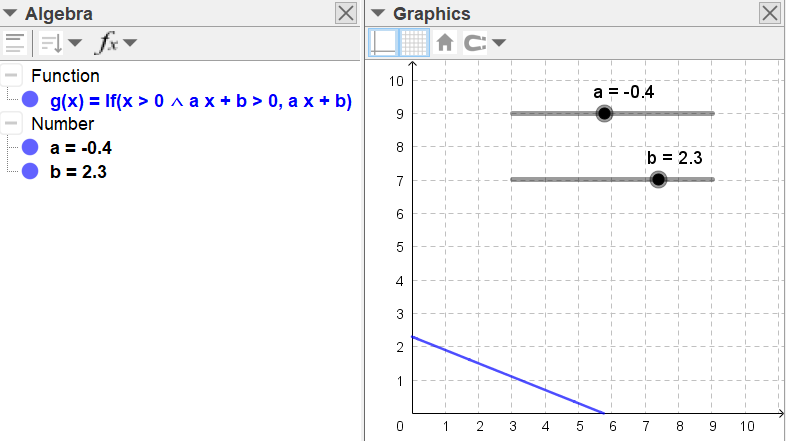# How to make a curve visible in only a certain quadrant?

StevenAC shared this question 2 years ago

I have the curve y = ax+b, with the values of a and b determined by sliders.

How can I make that curve visible in only a certain quadrant, like e.g. only left of the Y-axis and above the X-axis?2

With sliders a and b you can define If (x > 0 ∧ a x + b > 0, a x + b). Eventually you can choose to show the axes only in this quadrant too.

chris1

That worked perfectly, thanks! But now I want to do the same for a line that is not expressed as a mathematical formula (ax+b) but as a Geogebra expression (Line(A,B)). Apparently, a Geogebra expression is not accepted as a valid argument in the conditional set-up. Would you know the solution to this one too? Thanks in advance.2

eq1: LeftSide(Line(A, B)) - RightSide(Line(A, B)) + If(x ≥ 0 ∧ y ≥ 0, 0) = 0

pero yo pensaría si es suficiente hacer el segmento entre puntos de corte de la recta con los ejes1

excellent, thanks.